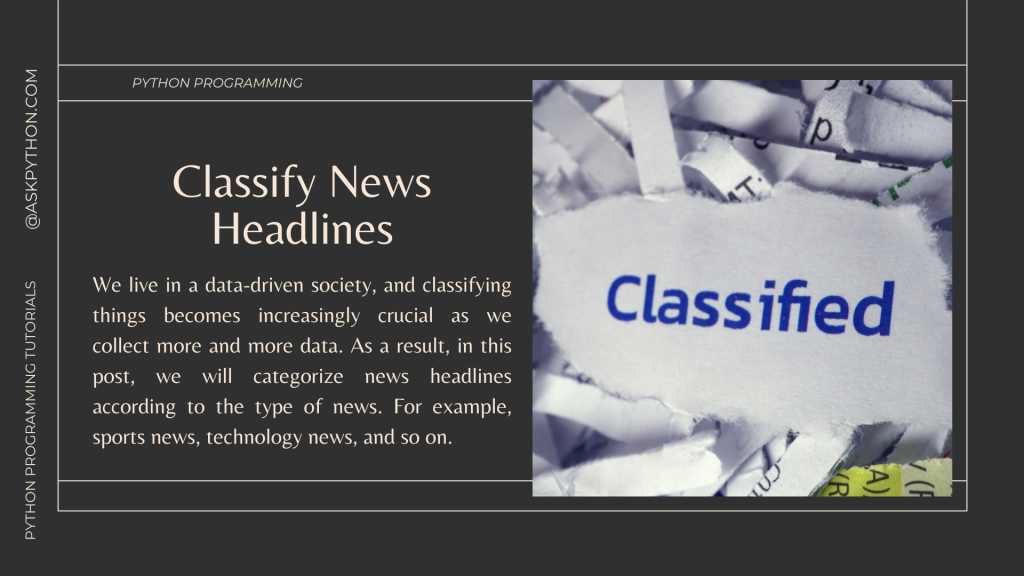# Classify News Headlines in Python – Machine LearningWe live in a data-driven society, and classifying things becomes increasingly crucial as we collect more and more data. As a result, in this post, we will categorize news headlines according to the type of news. For example, sports news, technology news, and so on.

In this tutorial, we would be working on data that will contain news headlines along with their category. Our objective would be to classify the news headlines by making use of the Machine Learning concepts in the Python programming language.

## Introducing the Dataset

We will use a dataset that includes news headlines along with their category. In this tutorial, we will not go into details like how web-scraping is done. You can download the dataset from here and then place it in your working directory.

## Steps to Classify News Headlines in Python

Let’s get into the steps that we’ll take to classify the news headlines in Python. Follow through with this tutorial to get an understanding of this entire process.

### 1. Importing Modules/Libraries

We’ll begin by importing the different modules that we’ll use. Copy-paste the below code snippet and proceed further.

```import tensorflow as tf
from tensorflow.keras import layers
from tensorflow.keras.models import Sequential
from tensorflow.keras.preprocessing.text import Tokenizer
from sklearn.model_selection import train_test_split
import numpy as np
import pandas as pd
```

```df = pd.read_csv('news_headlines.csv')
```

### 3. Train-Test Split

Now we would be doing the train-test split using the 80:20 rule where 80% of the data goes to training and the rest 20% goes to testing.

```training_data,testing_data =  train_test_split(df.iloc[:5000,:],test_size=0.2)
# 80% training data
```

To visualize things we can plot the training and testing separately with the help of the code mentioned below.

```import matplotlib.pyplot as plt
# plotting distribution of each news_category in training& testing data
plt.plot(training_data['news_category'].value_counts())
plt.plot(testing_data['news_category'].value_counts())
plt.title('Train-Test Split Visualization')
plt.show()
```

### 4. Tokenization Function

This function is quite simple and it takes place in the training and testing process of the data of the news headlines and to return sequences associated with them.

You may refer to this tutorial to understand more about the tokenization process.

```def tokenization_(training_headings, testing_headings, max_length=20,vocab_size = 5000):
tokenizer = Tokenizer(num_words = vocab_size, oov_token= '<oov>')

word_index = tokenizer.word_index

```

In order to apply the tokenizer function to the training and testing dataset, we would be required to run the following mentioned code snippet.

```tokenizer,X_train,X_test = tokenization_(training_data['news_headline'],

labels = {'sports':[0,1,0],'tech':[1,0,0],'world':[0,0,1],}
Y_train = np.array([labels[y] for y in training_data['news_category']])
Y_test = np.array([labels[y]  for y in testing_data['news_category'] ])
```

We would also be separating news_headline and their labels into different lists as they will be used in the model separately for training and testing purposes.

### 5. Building the Neural Network

```def build_model( n, vocab_size, embedding_size):
model = tf.keras.models.Sequential()
embedding_size,input_length=n))
metrics='accuracy')
print(model.summary())
return model
```

The code above does the following:

1. Create a sequential model
2. Add input and outpit layers to the sequential model
3. Compile the model and display the summary of the model after training
4. Finally, return the trained model

In this model, we will be making use of two layers where the first layer is an embedding layer and the second layer is the output layer.

### 6. Train the Neural Model

```epochs = 25
history = model.fit(X_train,Y_train,
validation_data = (X_test,Y_test),
epochs = epochs)
```

Initially, we will be set an epochs value. You can set it to whatever you prefer, for this model having 25 epochs will be enough. Next, we will be fitting our training and testing data into the neural model.

The model gave an accuracy of 97% on the training dataset and an accuracy of 94% on the validation/testing dataset which is pretty good and hence the model works pretty well.

## Conclusion

Congratulations! You just learned how to make a classification neural model in order to predict the category of news headlines. Hope you enjoyed it! 😇

Liked the tutorial? In any case, I would recommend you to have a look at the tutorials mentioned below:

Thank you for taking your time out! Hope you learned something new!! 😄